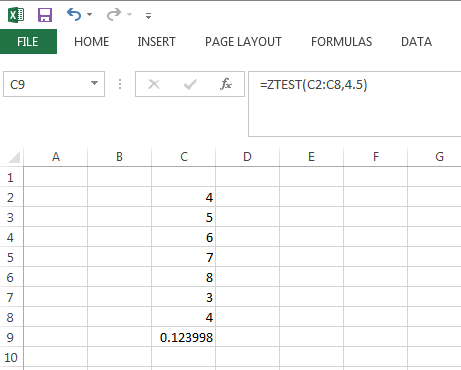# Porting Excel to .NET

In previous blog posts, we demonstrated calling NMath from within Excel (C#, Visual Basic). Another common use case is replacing an Excel spreadsheet with an equivalent .NET application. Today, we are releasing .NET code to make this task much easier.

We have created a library of Excel extensions for NMath that work just like the built-in Excel mathematical and statistical functions. They work with arrays in a similar fashion to a range of cells in Excel. Errors have been replaced with exceptions. Return values match as closely as possible.

For example, if you were using the `ZTest` function in Excel like so:This is how you would do the same thing in .NET with NMath:

```
using CenterSpace.NMath.Excel;

double[] array = { 4, 5, 6, 7, 8, 3, 4 };
ExcelStats.ZTest( array, 4.5 );```

That’s possible because we have code like this to make NMath work just like Excel:

```
public static double ZTest( double[] array, double mu, double sigma = Double.NaN )
{
if ( array.Length == 0 )
{
throw new ExcelNumException( "array must not be empty" );
}
sigma = Double.IsNaN( sigma ) ? StatsFunctions.StandardDeviation( array, BiasType.Unbiased ) : sigma;
var zTest = new OneSampleZTest( array, mu, sigma );
return zTest.P / 2.0;
}```

We have tested these functions against Excel directly and verified the results.

Here we provide an equivalent function to the MInverse (matrix inverse) function in Excel:

```
using CenterSpace.NMath.Excel;

public static double[,] MInverse( double[,] array )
{
if ( array.GetLength( 0 ) != array.GetLength( 1 ) )
{
throw new ExcelValueException( "array must be square" );
}
var matrix = new DoubleMatrix( array );
var factorization = new DoubleLUFact( matrix );
return factorization.Inverse().ToArray();
}```

In the case where you have a user-defined function that calls other built-in functions, you can replace your code easily. For example, a user-defined function as follows:

```Function CV(numbers As Range)
mean = Application.WorksheetFunction.Average(numbers)
standarddeviation = Application.WorksheetFunction.StDev(numbers)
CV = (standarddeviation / mean) * 100
End Function
```

would look as follows:

```    private static double CV( double[] a )
{
var mean = ExcelStats.Average( a );
var stddev = ExcelStats.StDev( a );
return ( stddev / mean ) * 100.0;
}
```

To use this functionality:

1. Download the free trial versions of our NMath and NMath Stats libraries. Trial versions are distributed in distributed in binary form only for a 30-day evaluation period. If you are new to NMath, you may find our Getting Started video helpful.
2. Download the Excel extension library here. It comes with source code, so you can see exactly how Excel functions are implement in NMath. (In a future release, this functionality will be built into NMath.)

Note: The Excel extensions are designed as static methods, allowing a one-to-one mapping from Excel to NMath. In some cases, such as within loops, greater performance can be achieved by maintaining NMath state between calls.

– Trevor

## Porting Excel to .NET

Below is a complete list of supported Excel functions which are now directly supported in NMath.

```ACos
ACosH
ASin
ASinH
ATan
ATan2
ATanH
Abs
AveDev
Average
BetaInv
BinomDist
Ceiling
ChiDist
ChiInv
Combin
Confidence
Correl
Cos
CosH
Count
CountBlank
CountIf
Covar
CritBinom
Degrees
DevSq
Even
Exp
ExponDist
FDist
FInv
FTest
Fact
FactDouble
Floor
Forecast
Frequency
GCD
GammaInv
GammaLn
GeoMean
Growth
HarMean
Int
Intercept
Kurt
Large
LinEst
Ln
Log
Log10
LogEst
LogNormDist
MDeterm
MInverse
MMult
Max
Median
Min
Mod
Mode
NegBinomDist
NormDist
NormInv
NormSDist
NormSInv
Odd
Pearson
PercentRank
Percentile
Permut
Pi
Poisson
Power
Prob
Product
Quartile
Quotient
Rand
RandBetween
Rank
Round
RoundDown
RoundUp
Sign
Sin
SinH
Skew
Slope
Small
SqRt
SqRtPi
StDev
StDevP
StEYX
Standardize
Sum
SumSq
TDist
TInv
TTest
Tan
TanH
Trend
TrimMean
Var
VarP
Weibull
ZTest```
Top